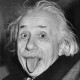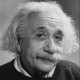# Concinnitas: The Art of the Equation

•Matteo Cantiello
•Alberto Pepe
•Albert Einstein
•Albert Einstein

San Francisco, CA – On view at Crown Point Press is an exhibition of etchings by scientists and mathematicians, September 4 - October 27, 2015.

We came across this set of beautiful etchings on Artsy depicting mathematical equations. We decided to reproduce them on Authorea, using our equation editor and some LaTeX. Here’s the result.

\begin{equation} \frac{\mathscr{d}}{\mathscr{dA}}\int_{\mathcal{G}}\rho\mathscr{dV}=-\int_{\partial\mathcal{G}}\mathscr{f}\cdot\mathscr{n}\,\mathscr{dS}\nonumber \\ \end{equation}Conservation Laws by Peter Lax, 2014

\begin{equation} \mathcal{O}_{\mathscr{m_{g}}}(\kappa)\cong\big{(}\Lambda^{\mathscr{g}}\pi_{*}\,\omega_{\mathscr{e_{g}/m_{g}}}\big{)}^{\otimes\mathscr{13}}\nonumber \\ \end{equation}Thirteen?? by David Mumford, 2014

\begin{equation} \mathcal{Sign}\,(y)-\mathop{{{\int}}}_{y}L_{\kappa}\big{(}\,p(y)\big{)}=(-1)^{\kappa+1}\,\eta(A)\nonumber \\ \end{equation}The Index Theorem by Michael Atiyah, 2014

\begin{equation} \mathcal{T}(\mathcal{n})=\sum\frac{(a-b)(a-c)(a-d)(a-e)(b-c)(b-d)(b-e)(c-d)(c-e)(d-e)}{1\,!\,2\,!\,3\,!\,4\,!}\nonumber \\ \end{equation}The MacDonald Equation by Freeman Dyson, 2014